# How to use the MAX function in Excel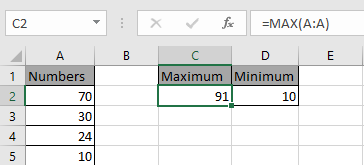In this article, we will learn How to use the MAX function in Excel.

MAXIMUM or largest value in array

IN Excel, many times we need the lowest or the highest value present in the list. For example finding the largest or maximum sales value in employees dataset. Or finding the largest discount percentage in the products table. Let's understand the function syntax and an example to illustrate the function usage.

MAX Function in Excel

MAX function returns the maximum number is the range.

Syntax:

 =MAX(array)

array : list of numbers given as array reference in the function. Like values in A1 to A75 be referred as A1:A75

Example :

All of these might be confusing to understand. Let's understand how to use the function using an example. Maximum of two input numbers is done via Formula shown below.

Use the formula

 =MAX(20,21)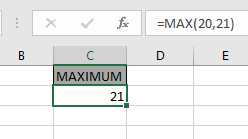Now we wish to find the Maximum from the entire column.

Here we have 76 numbers in column A.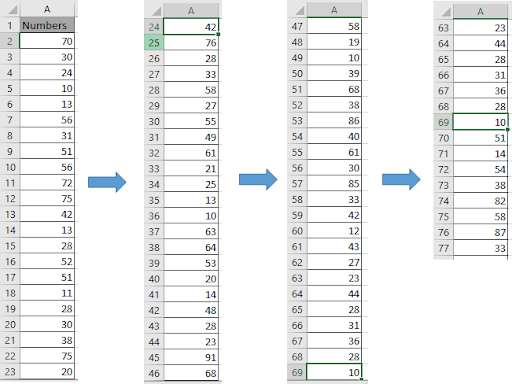Here we need to Maximum value present in this column. There would two methods

Use the formula

 =MAX(A2:A77) OR =MAX(A:A)As you can see you can get the maximum value from the range of numbers. You can get the minimum value from the range using the MIN function in Excel.

MAXA function in Excel

MAXA function is a mathematical function which returns the numerical largest value from an array of numbers given in as input. The MAXA function considers logic values also whereas MAX function doesn't. MAXA function given a list of values as arguments returns the maximum numeric value in the cell.

MAXA function Syntax:

 =MAXA(number1,number2,..)

number : number or array given as cell reference

MAXA function Example:

All of these might be confusing to understand. So, let's test this formula via running it on the example shown below. Here we have some numbers to test the MAXA function in Excel.

Use the formula:

 =MAXA(C1:C17)

Here the array of values is given as cell reference. You can also input values as numbers.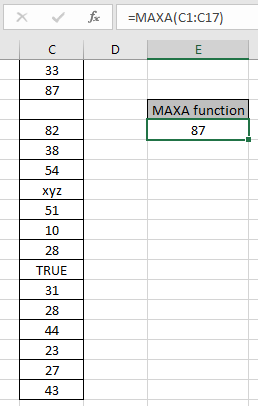Here the function ignores blank cells, TRUE logic value is considered as 1 & xyz text as zero. The function returns 87 as numerically the largest value from the array C1:C17.

Now consider one more example of MAXA function:

Here we take negative numbers and Logic value in array and take the respective from both MAXA and MAX function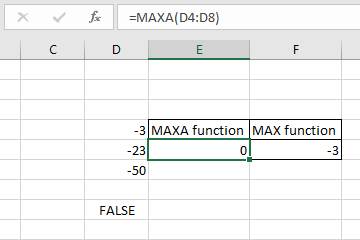-3 is the numerically the largest value so the MAX function    ignores logic values and returns -3 but MAXA considers TRUE as 1 and FALSE as 0 numeric value and returns 0 as result.

Here are some observational notes using the MAX function in Excel shown below.

Notes:

1. The formula only works with numbers and text.
2. The MAXA function considers logic values TRUE as 1 and FALSE as 0 whereas MAX function ignores.
3. The function takes 0 if no number is given in as input.

Hope this article about How to use the MAX function in Excel is explanatory. Find more articles on calculating values and related Excel formulas here. If you liked our blogs, share it with your friends on Facebook. And also you can follow us on Twitter and Facebook. We would love to hear from you, do let us know how we can improve, complement or innovate our work and make it better for you. Write to us at info@exceltip.com.

Related Articles :

How to use the MAX function in Excel | returns the numerical largest value from the given array using the MAX function in Excel

How to use the MIN function in Excel | returns the numerical smallest value from the given array using the MIN function in Excel

How to use the LARGE function in Excel | returns the numerical largest nth value from the given array using the LARGE function in Excel

How to use the ISEVEN function in Excel | returns the TRUE logic value if the number is MAX using the ISEVEN function in Excel.

How to use the ISODD function in Excel | returns the TRUE logic value if the number is ODD using the ISODD function in Excel.

Calculate max if condition match : Get the MAX value from the array having criteria.

How to Use Excel AGGREGATE Function : Use the AGGREGATE function to perform multiple operation with multiple criterias in excel.

COUNTIFS With OR For Multiple Criteria : Count cells having multiple criteria match using the OR function.

How to use wildcards in excel : Count cells matching phrases using the wildcards in excel

Popular Articles :

How to use the IF Function in Excel : The IF statement in Excel checks the condition and returns a specific value if the condition is TRUE or returns another specific value if FALSE.

How to use the VLOOKUP Function in Excel : This is one of the most used and popular functions of excel that is used to lookup value from different ranges and sheets.

How to use the SUMIF Function in Excel : This is another dashboard essential function. This helps you sum up values on specific conditions.

How to use the COUNTIF Function in Excel : Count values with conditions using this amazing function. You don't need to filter your data to count specific values. Countif function is essential to prepare your dashboard.

Terms and Conditions of use

The applications/code on this site are distributed as is and without warranties or liability. In no event shall the owner of the copyrights, or the authors of the applications/code be liable for any loss of profit, any problems or any damage resulting from the use or evaluation of the applications/code.